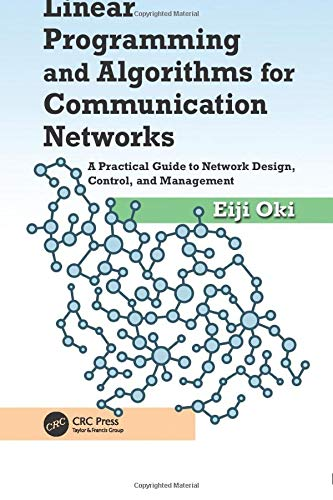# Linear Programming and Algorithms for Communication Networks: A Practical Guide to Network Design, Control, and ManagementPrice: ₹3,634.00
(as of Dec 08,2021 13:38:55 UTC – Details)Explaining how to apply to mathematical programming to network design and control, Linear Programming and Algorithms for Communication Networks: A Practical Guide to Network Design, Control, and Management fills the gap between mathematical programming theory and its implementation in communication networks. From the basics all the way through to more advanced concepts, its comprehensive coverage provides readers with a solid foundation in mathematical programming for communication networks.

Addressing optimization problems for communication networks, including the shortest path problem, max flow problem, and minimum-cost flow problem, the book covers the fundamentals of linear programming and integer linear programming required to address a wide range of problems. It also:

• Examines several problems on finding disjoint paths for reliable communications
• Addresses optimization problems in optical wavelength-routed networks
• Describes several routing strategies for maximizing network utilization for various traffic-demand models
• Considers routing problems in Internet Protocol (IP) networks
• Presents mathematical puzzles that can be tackled by integer linear programming (ILP)

Using the GNU Linear Programming Kit (GLPK) package, which is designed for solving linear programming and mixed integer programming problems, it explains typical problems and provides solutions for communication networks. The book provides algorithms for these problems as well as helpful examples with demonstrations. Once you gain an understanding of how to solve LP problems for communication networks using the GLPK descriptions in this book, you will also be able to easily apply your knowledge to other solvers.

Scroll to Top